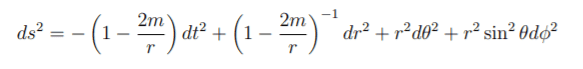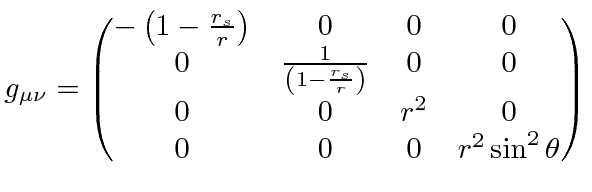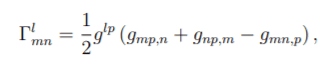# Trying To Calculate Christoffel Symbols

cgreeleybsu
I am trying to create a function to calculate the Christoffel Symbols of a given metric (in this case the Shwartzchild metric). Calculating the (non zero) Christoffel Symboles for the Shwartzchild connection, I am a double major in Physics and Computer Science so I decided to go the code rout. It looked pretty trivial but I appear to be getting the wrong answers and I am trying to figure out why. I am wondering if maybe you can't address the indices in the way I am, but I don't know how you would otherwise, or maybe I am taking the tensor product incorrectly?

If anyone could review what I have done and give me some pointers that would be great! I have it running in a Jupyter Notebook but I will just post the code here as a python file. The debug option will output the entire Christoffel tensor as XML so you can copy/paste it into a text editor like notepad++ and fold the different parts up.

I am uploading the python code as a .txt just rename to .py (or copy/paste into one) when downloaded please :) numpy, scipy, and sympy packages are required

NOTE 0: I am not asking for help with debugging, I am trying to figure out mathematically what I am doing wrong in the code.

NOTE 1: Actual calculation at line 77

- Chris

P.s IntermediateConnection was just another attempt at calculating this, I haven't touched it in a while.

#### Attachments

• Connection.txt
6.3 KB · Views: 159

Mentor
While I don't know what's wrong, I did notice your use of debug prints.

Have you looked at PyCharm? It has an excellent debugging tool that would allow to step through the code and look at values meaning you can clean out all those debug prints.

https://www.jetbrains.com/pycharm/

cgreeleybsu
While I don't know what's wrong, I did notice your use of debug prints.

Have you looked at PyCharm? It has an excellent debugging tool that would allow to step through the code and look at values meaning you can clean out all those debug prints.

https://www.jetbrains.com/pycharm/

Thanks jedishrfu, I might take a look, however am trying to figure out if the problem here that I am having is less a bug with the code and more a bug in the way I am trying to do my math? I don't know if a step by step debugger will help me with that 🤷‍♂️ but I may give it a try. Basically I have 4 nested for loops one for the index at the top of the Christoffel symbol (for each matrix in it), 2 for the rows and columbs of the matracies and one independent index. I iterate through them in that order so it looks like...

Christoffel( l, m, n ), where l is the current matrix, m is the row, n is the columb, and p is the independant index, thought all these numbers are moved around to index different things at different points and do not strictly represent the matrix/row/coumb, etc. throughout the calculation (the number next to each letter represents the number that the index called by that letter will be at at that time):

l0:
• m0:
• n0:
• p0 p1 p2 p3
• n1:
• p0 p1 p2 p3
• n2:
• p0 p1 p2 p3
• n3:
• p0 p1 p2 p3
• m1:
• n0:
• p0 p1 p2 p3
• n1:
• p0 p1 p2 p3
• n2:
• p0 p1 p2 p3
• n3:
• p0 p1 p2 p3
• m2:
• n0:
• p0 p1 p2 p3
• n1:
• p0 p1 p2 p3
• n2:
• p0 p1 p2 p3
• n3:
• p0 p1 p2 p3
• m3:
• n0:
• p0 p1 p2 p3
• n1:
• p0 p1 p2 p3
• n2:
• p0 p1 p2 p3
• n3:
• p0 p1 p2 p3
l1:
• m0:
• n0:
• p0 p1 p2 p3
• n1:
• p0 p1 p2 p3
• n2:
• p0 p1 p2 p3
• n3:
• p0 p1 p2 p3
• m1:
• n0:
• p0 p1 p2 p3
• n1:
• p0 p1 p2 p3
• n2:
• p0 p1 p2 p3
• n3:
• p0 p1 p2 p3
• m2:
• n0:
• p0 p1 p2 p3
• n1:
• p0 p1 p2 p3
• n2:
• p0 p1 p2 p3
• n3:
• p0 p1 p2 p3
• m3:
• n0:
• p0 p1 p2 p3
• n1:
• p0 p1 p2 p3
• n2:
• p0 p1 p2 p3
• n3:
• p0 p1 p2 p3
l2:
• m0:
• n0:
• p0 p1 p2 p3
• n1:
• p0 p1 p2 p3
• n2:
• p0 p1 p2 p3
• n3:
• p0 p1 p2 p3
• m1:
• n0:
• p0 p1 p2 p3
• n1:
• p0 p1 p2 p3
• n2:
• p0 p1 p2 p3
• n3:
• p0 p1 p2 p3
• m2:
• n0:
• p0 p1 p2 p3
• n1:
• p0 p1 p2 p3
• n2:
• p0 p1 p2 p3
• n3:
• p0 p1 p2 p3
• m3:
• n0:
• p0 p1 p2 p3
• n1:
• p0 p1 p2 p3
• n2:
• p0 p1 p2 p3
• n3:
• p0 p1 p2 p3
l3:
• m0:
• n0:
• p0 p1 p2 p3
• n1:
• p0 p1 p2 p3
• n2:
• p0 p1 p2 p3
• n3:
• p0 p1 p2 p3
• m1:
• n0:
• p0 p1 p2 p3
• n1:
• p0 p1 p2 p3
• n2:
• p0 p1 p2 p3
• n3:
• p0 p1 p2 p3
• m2:
• n0:
• p0 p1 p2 p3
• n1:
• p0 p1 p2 p3
• n2:
• p0 p1 p2 p3
• n3:
• p0 p1 p2 p3
• m3:
• n0:
• p0 p1 p2 p3
• n1:
• p0 p1 p2 p3
• n2:
• p0 p1 p2 p3
• n3:
• p0 p1 p2 p3

It seems like iterating through the indices and summing everything at all combinations of l, m, and n (after running through 0 through 3 on p) would be right and basically a quadruple sigma, but I'm getting the wrong results, I don't know if this is the source of my error or not and if I am doing the Einstien summation correctly or if it is something else? 🤷‍♂️

Last edited:
Mentor
What I would do is have the program print out the indices and calculations it's doing so that you can verify the order is correct. I assuming you understand what you are attempting to calculate. It looks like your code is doing that.

What is the actual expression mathematically that is using Einstein summation?

Mentor
As an example, given a tensor product like:

##C^i_k = A^i_j * B^j_k##

Python:
for i in range(4):
for k in range(4):
for j in range(4):
C [ i ][ k ] = C [ i ][ k ] + A [ i ][ j ] *  B [ j ] [ k ]

cgreeleybsu
What I would do is have the program print out the indices and calculations it's doing so that you can verify the order is correct. I assuming you understand what you are attempting to calculate. It looks like your code is doing that.

What is the actual expression mathematically that is using Einstein summation?

"g" or in the code "metric" is a 4x4 matrix with the things in front of the 'd' whatevers in this equation(source: my homework)
along the diangnal too look like this(source: https://hepweb.ucsd.edu/ph110b/110b_notes/img1109.png)

Then the actual expression I am trying to calculate is this(source: my homework)

a 4x4x4 "3d matrix"

Staff Emeritus
Homework Helper
Gold Member
The debug option will output the entire Christoffel tensor
Just to interject something that is vital for understanding differential geometry: The Christoffel symbols are not the components of a tensor field. They are the connection coefficients of the Levi-Civita connection.

•vanhees71 and jedishrfu
Mentor
Given what you wrote you loops should follow like this:

Python:
for l in range(4):
for m in range(4):
for n in range(4):
for p in range(4):
GAMMA [ l ] [ m ] [ n ] = GAMMA [ l ] [ m ] [ n ] + ...your expression in l, m, n, and p...

Alternatively, you could replace the GAMMA with GAMOW in honor of Prof George Gamow.

** In general, I don't like to use l as a variable as its easy to confuse it with ONE. The same goes for O vs ZERO.

Also, I found this reference on tensor notation that may be of interest to you. There is an example very similar to the one I gave earlier for summing it on a computer.

https://www.continuummechanics.org/tensornotationbasic.html

cgreeleybsu
Given what you wrote you loops should follow like this:

Python:
for l in range(4):
for m in range(4):
for n in range(4):
for p in range(4):
GAMMA [ l ] [ m ] [ n ] = GAMMA [ l ] [ m ] [ n ] + ...your expression in l, m, n, and p...

Thats about what my code looks like.

Alternatively, you could replace the GAMMA with GAMOW in honor of Prof George Gamow.

** In general, I don't like to use l as a variable as its easy to confuse it with ONE. The same goes for O vs ZERO.

Fair, it was just the source I was looking at at the time, used the same letters to make it easier.

Also, I found this reference on tensor notation that may be of interest to you. There is an example very similar to the one I gave earlier for summing it on a computer.

https://www.continuummechanics.org/tensornotationbasic.html

I looked there, I tried modifying my code by changing:
Code:
results[ l ][ m ][ n ] += ( 0.5 * metric[ l ][ p ] *
( Derive( metric, m, p, symboles[ n ] ) +
Derive( metric, n, p, symboles[ m ] ) -
Derive( metric, m, n, symboles[ p ] ) ) )

to

Code:
results[ l ][ m ][ n ] += ( ( Derive( metric, m, p, symboles[ n ] ) +
Derive( metric, n, p, symboles[ m ] ) -
Derive( metric, m, n, symboles[ p ] ) ) )

Code:
            for i in range( 4 ):
results[ i ] = ( 0.5 * Matrix( metric ) * Matrix( results[ i ] ) ).tolist()

after everything was summed and calculated, basically calculating the matricies (adding/subtracting) then multiplying them by the plain metric using the Matrix class to avoid error, this should work in this case, ran a quick test too see if:
A*B + A*C = A( B + C )
and it seemed too. However what I got out was a bunch of garbdly gook, so it makes me question either that premise or my calculations.

I question the ladder, it would seem that
Code:
metric[ l ][ p ]
in
Code:
metric[ l ][ p ] * ( Derive( metric, m, p, symboles[ n ] ) + Derive( metric, n, p, symboles[ m ] ) - Derive( metric, m, n, symboles[ p ] ) ) )
only goes up and down one row for each matrix in the resulting tensor, I don't know if that is something that is suppose to happen or not? The results seem more correct than a straight up multiplication. I just don't know if this is a valid way to multiply a matrix/take a tensor product(?)

As an example, given a tensor product like:

##C^i_k = A^i_j * B^j_k##

Python:
for i in range(4):
for k in range(4):
for j in range(4):
C [ i ][ k ] = C [ i ][ k ] + A [ i ][ j ] *  B [ j ] [ k ]

I'm confused by this, shouldn't at least one dimension change?

Last edited:
cgreeleybsu
Just to interject something that is vital for understanding differential geometry: The Christoffel symbols are not the components of a tensor field. They are the connection coefficients of the Levi-Civita connection.

I realize this may be exactly what you are trying to contradict, but as I understand (or don't) right now, the components of Christoffel symbols are matrices, is this incorrect?

I assume that is what you are saying by saying they are not components of a tensor field (i.e the tensor @ X point is Y).

Is this because my understanding above is incorrect, or because Christoffel symbols are part of Riemann Tensors and the Riemann Tensors are what make up the tensor field?

Staff Emeritus
Homework Helper
Gold Member
I realize this may be exactly what you are trying to contradict, but as I understand (or don't) right now, the components of Christoffel symbols are matrices, is this incorrect?

I assume that is what you are saying by saying they are not components of a tensor field (i.e the tensor @ X point is Y).

Is this because my understanding above is incorrect, or because Christoffel symbols are part of Riemann Tensors and the Riemann Tensors are what make up the tensor field?
A tensor field in this context is not a matrix. It may be represented by an N-dimensional array of numbers or an N-dimensional array of numbers may define the components of a tensor in a particular coordinate system. However, tensor components in different systems have very particular relationships with each other. Those relationships are not satisfied by the Christoffel symbols, which therefore cannot be the components of a tensor field.

The Riemann tensor is a tensor field whose components can be expressed in terms of the Christoffel symbols. However, the Christoffel symbols then appear in a very particular combination that does satisfy the correct coordinate transformation rules. The Riemann tensor is far from the only tensor in GR.

Mentor
I'm confused by this, shouldn't at least one dimension change?
In this example for a given i,k index we are summing over the j elements and since we need all values for C then we index over i and k too.

cgreeleybsu
A tensor field in this context is not a matrix.
I think I was trying to say is the Christoffel symbol is composed of matracies, in otherwords n*m*l

It may be represented by an N-dimensional array of numbers or an N-dimensional array of numbers may define the components of a tensor in a particular coordinate system. However, tensor components in different systems have very particular relationships with each other. Those relationships are not satisfied by the Christoffel symbols, which therefore cannot be the components of a tensor field.
I think you answered my third question, thank you

The Riemann tensor is a tensor field whose components can be expressed in terms of the Christoffel symbols. However, the Christoffel symbols then appear in a very particular combination that does satisfy the correct coordinate transformation rules. The Riemann tensor is far from the only tensor in GR.

Thank you, I do know its not the only tensor, I didnt know about the "appear in a very particular combination that does satisfy the correct coordinate transformation rules" thing, that's interesting.

Gold Member
2022 Award
I realize this may be exactly what you are trying to contradict, but as I understand (or don't) right now, the components of Christoffel symbols are matrices, is this incorrect?

I assume that is what you are saying by saying they are not components of a tensor field (i.e the tensor @ X point is Y).

Is this because my understanding above is incorrect, or because Christoffel symbols are part of Riemann Tensors and the Riemann Tensors are what make up the tensor field?
It's just important to keep in mind that under general changes of the coordinates the Christoffel symbold do not transform as tensor components do.

It's also important to keep in mind that physicists are often saying "tensor" but mean "tensor components". Of course a tensor doesn't depend on the choice of coordinates at all. It's an invariant object. That's the whole point of having the physical laws formulated as laws between tensors (or tensor fields).

•cgreeleybsu, Orodruin and jedishrfu
cgreeleybsu
It's just important to keep in mind that under general changes of the coordinates the Christoffel symbold do not transform as tensor components do.

It's also important to keep in mind that physicists are often saying "tensor" but mean "tensor components". Of course a tensor doesn't depend on the choice of coordinates at all. It's an invariant object. That's the whole point of having the physical laws formulated as laws between tensors (or tensor fields).
Thank you, and sorry I haven't replied to this question in a bit --life stuff--

I haven't figured this out yet though.

Gold Member
2022 Award
Then I recommend to read the GR part of Landau&Lifshitz vol. 2. There they develop the tensor calculus (in the Ricci calculus) along with the physics in a very nice way.

Staff Emeritus
•